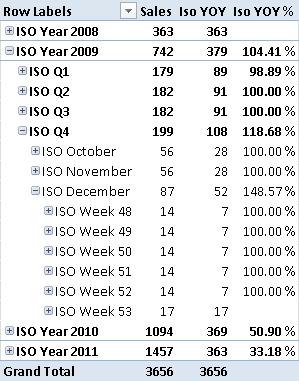In the Week-Based Time Intelligence in DAX article, I described how to implement time intelligence calculations in DAX that works on ISO calendars. This approach offers a simplified way to implement custom time related calculations in DAX, as shown in the Time Patterns article on DAX Patterns web site.

In both cases, the Year-Over-Year (YOY) calculation assumes that you can obtain the corresponding period in the previous year through a simple DAX formula. This assumption might be hard to handle when there are exceptions in data, such as the 53rd week in an ISO Calendar that appears only in some years. The YOY based on the relative week number within the year produces a comparison with a week that has no sales. In the following example, the YOY of Week 52 in 2009 has the same value of Sales, because there is no week 53 in 2008.Looking at the content of the Dates date, you can see that the week 53 of 2009 starts on Dec. 28, 2009, and ends on Jan 3, 2010. 2008, on the other hand, has only 52 weeks.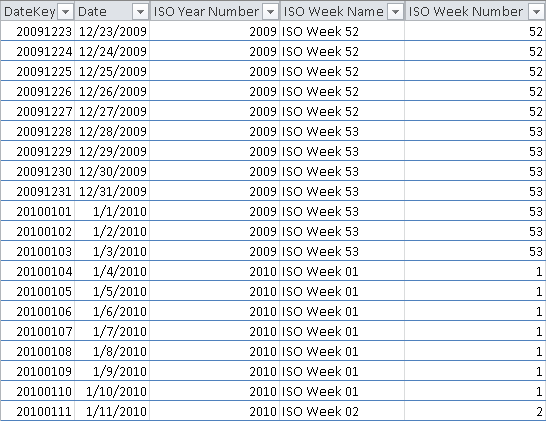What is the week to use as a YOY comparison term for week 53 in 2009? There could be many answers to this question. The year 2008 has only 52 weeks, so you might use the last week in 2008 (comparing both W52 and W53 of 2009 with the same W52 in 2008), or you might use the first week in 2009 (comparing W53-2009 with W01-2009). Whatever you business requirements are, you need to understand that the exception is in the data, not in the formula. Thus, you cannot (and should not) handle the exception using some DAX code. What you need to do is shape your data so that it handles the exception.

In order to remove such a logic from the DAX code, a good approach is to create a column containing, for each day, the corresponding day in the previous year to use for YOY comparison. This is the purpose of the ISO YOY Date column, which you can see in the following picture. In this example, we chose to compare W53-2009 with W01-2009, repeating the same week as a comparison term for W01-2010.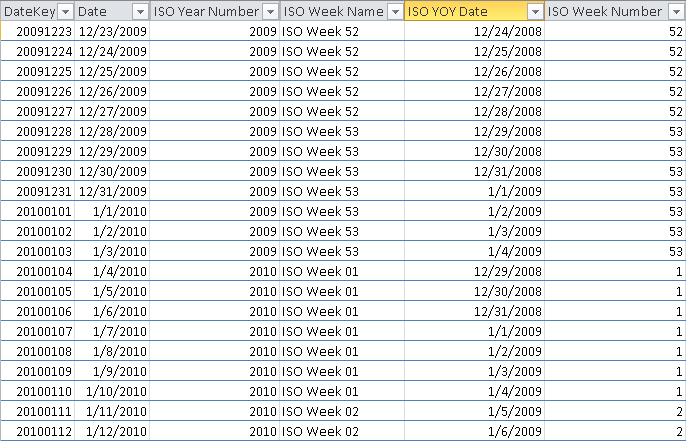The date range Dec. 29, 2008 to Jan 4, 2009 corresponds to the first week in 2009, as you can see in the following picture.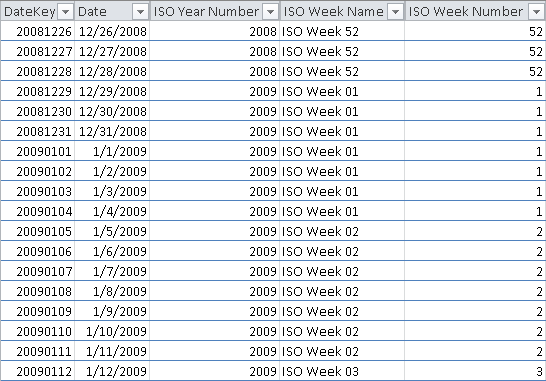At this point, you can write the following DAX implementation.

```ISO PY Custom :=
CALCULATE (
SUM ( Sales[Sales Amount] ),
FILTER (
ALL ( Dates ),
CONTAINS (
VALUES ( Dates[ISO YOY Date] ),
Dates[ISO YOY Date],
Dates[Date]
)
)
)

Iso YOY Custom := [Sales] - [ISO PY Custom]

Iso YOY Custom % :=
IF (
[Iso PY Custom] <> 0,
[Iso YOY Custom] / [Iso PY Custom],
BLANK()
)
```

The idea behind the ISO PY Custom measure is to shift the filter of Dates back of one year, based on the current selection of Dates. The CONTAINS function filters the dates corresponding to the current selection of values in the ISO YOY Date column. Such a technique “translates” that set of dates in a new filter used by the CALCULATE statement.

You can see the result of Iso YOY Custom measure in the following picture.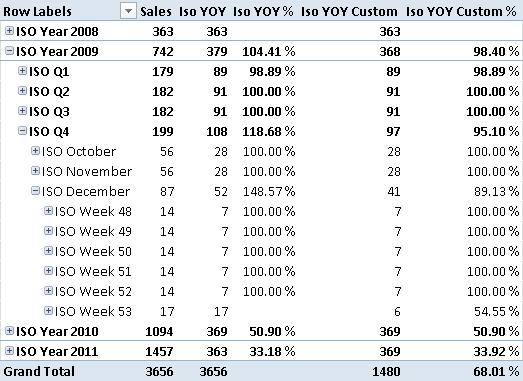This same approach is useful every time you want to implement a custom “shift” logic to compare the current selection of dates.

CONTAINSReturns TRUE if there exists at least one row where all columns have specified values.

`CONTAINS ( <Table>, <ColumnName>, <Value> [, <ColumnName>, <Value> [, … ] ] )`

CALCULATEContext transition

Evaluates an expression in a context modified by filters.

`CALCULATE ( <Expression> [, <Filter> [, <Filter> [, … ] ] ] )`